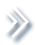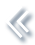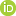####ArchivesReview Articles

## An equivalence result for moment equations when data are missing at random

Marian Hristache ,

Univ Rennes, Ensai, CNRS, CREST-UMR 9194, Rennes, FranceValentin Patilea

Univ Rennes, Ensai, CNRS, CREST-UMR 9194, Rennes, Francevalentin.patilea@ensai.fr

Pages 199-207 | Received 19 Dec. 2018, Accepted 21 Sep. 2019, Published online: 09 Oct. 2019,
• Abstract
• References
• Citations

ABSTRACT

We consider general statistical models defined by moment equations when data are missing at random. Using the inverse probability weighting, such a model is shown to be equivalent with a model for the observed variables only, augmented by a moment condition defined by the missing mechanism. Our framework covers a large class of parametric and semiparametric models where we allow for missing responses, missing covariates and any combination of them. The equivalence result is stated under minimal technical conditions and sheds new light on various aspects of interest in the missing data literature, as for instance the efficiency bounds and the construction of the efficient estimators, the restricted estimators and the imputation.

## References

1. Ai, C., & Chen, X. (2003). Efficient estimation of models with conditional moment restrictions containing unknown functions. Econometrica71, 1795–1843. doi: 10.1111/1468-0262.00470 [Crossref][Web of Science ®], [Google Scholar]
2. Ai, C., & Chen, X. (2007). Estimation of possibly misspecified semiparametric conditional moment restriction models with different conditioning variables. Journal of Econometrics141, 5–43. doi: 10.1016/j.jeconom.2007.01.013 [Crossref][Web of Science ®], [Google Scholar]
3. Ai, C., & Chen, X. (2012). The semiparametric efficiency bound for models of sequential moment restrictions containing unknown functions. Journal of Econometrics170, 442–457. Thirtieth Anniversary of Generalized Method of Moments. doi: 10.1016/j.jeconom.2012.05.015 [Crossref][Web of Science ®], [Google Scholar]
4. Chen, X., Hong, H., & Tarozzi, A. (2008). Semiparametric efficiency in GMM models with auxiliary data. The Annals of Statistics36, 808–843. doi: 10.1214/009053607000000947 [Crossref][Web of Science ®], [Google Scholar]
5. Chen, S. X., & Van Keilegom, I. (2013). Estimation in semiparametric models with missing data. Annals of the Institute of Statistical Mathematics65, 785–805. doi: 10.1007/s10463-012-0393-6 [Crossref][Web of Science ®], [Google Scholar]
6. Chen, X., Wan, A. T. K., & Zhou, Y. (2014). Efficient quantile regression analysis with missing observations. Journal of the American Statistical Association110(510), 723–741. doi: 10.1080/01621459.2014.928219 [Taylor & Francis Online][Web of Science ®], [Google Scholar]
7. Chen, X., Wan, A. T. K., & Zhou, Y. (2015). Efficient quantile regression analysis with missing observations. Journal of the American Statistical Association110, 723–741. doi: 10.1080/01621459.2014.928219 [Taylor & Francis Online][Web of Science ®], [Google Scholar]
8. Cheng, P. E. (1994). Nonparametric estimation of mean functionals with data missing at random. Journal of the American Statistical Association89, 81–87. doi: 10.1080/01621459.1994.10476448 [Taylor & Francis Online][Web of Science ®], [Google Scholar]
9. Domínguez, M. A., & Lobato, I. N. (2004). Consistent estimation of models defined by conditional moment restrictions. Econometrica72, 1601–1615. doi: 10.1111/j.1468-0262.2004.00545.x [Crossref][Web of Science ®], [Google Scholar]
10. Graham, B. S. (2011). Efficiency bounds for missing data models with semiparametric restrictions. Econometrica79, 437–452. doi: 10.3982/ECTA7379 [Crossref][Web of Science ®], [Google Scholar]
11. Heitjan, D. F., & Rubin, D. B. (1991). Ignorability and coarse data. The Annals of Statistics19, 2244–2253. doi: 10.1214/aos/1176348396 [Crossref][Web of Science ®], [Google Scholar]
12. Hristache, M., & Patilea, V. (2016). Semiparametric efficiency bounds for conditional moment restriction models with different conditioning variables. Econometric Theory32, 917–946. doi: 10.1017/S0266466615000080 [Crossref][Web of Science ®], [Google Scholar]
13. Hristache, M., & Patilea, V. (2017). Conditional moment models with data missing at random. Biometrika104, 735–742. doi: 10.1093/biomet/asx025 [Crossref][Web of Science ®], [Google Scholar]
14. Lavergne, P., & Patilea, V. (2013). Smooth minimum distance estimation and testing with conditional estimating equations: uniform in bandwidth theory. Journal of Econometrics177, 47–59. doi: 10.1016/j.jeconom.2013.05.006 [Crossref][Web of Science ®], [Google Scholar]
15. Little, R., & Rubin, D. (2002). Statistical analysis with missing data. Wiley series in probability and mathematical statistics. Probability and mathematical statistics. John Wiley & Sons, Inc., Hoboken, New Jersey. [Google Scholar]
16. Müller, U. U. (2009). Estimating linear functionals in nonlinear regression with responses missing at random. The Annals of Statistics37, 2245–2277. doi: 10.1214/08-AOS642 [Crossref][Web of Science ®], [Google Scholar]
17. Prokhorov, A., & Schmidt, P. (2009). GMM redundancy results for general missing data problems. Journal of Econometrics151, 47–55. doi: 10.1016/j.jeconom.2009.03.010 [Crossref][Web of Science ®], [Google Scholar]
18. Robins, J. M., & Gill, R. D. (1997). Non-response models for the analysis of non-monotone ignorable missing data. Statistics in Medicine16, 39–56. doi: 10.1002/(SICI)1097-0258(19970115)16:1<39::AID-SIM535>3.0.CO;2-D [Crossref][Web of Science ®], [Google Scholar]
19. Rosenbaum, P. R., & Rubin, D. B. (1983). The central role of the propensity score in observational studies for causal effects. Biometrika70, 41–55. doi: 10.1093/biomet/70.1.41 [Crossref][Web of Science ®], [Google Scholar]
20. Rubin, D. B. (1976). Inference and missing data. Biometrika63, 581–592. doi: 10.1093/biomet/63.3.581 [Crossref][Web of Science ®], [Google Scholar]
21. Tan, Z. (2011). Efficient restricted estimators for conditional mean models with missing data. Biometrika98, 663–684. doi: 10.1093/biomet/asr007 [Crossref][Web of Science ®], [Google Scholar]
22. Tsiatis, A. (2007). Semiparametric theory and missing data. New York: Springer-Verlag. [Google Scholar]
23. van der Laan, M. J., & Robins, J. M. (2003). Unified methods for censored longitudinal data and causality. New York: Springer-Verlag. [Crossref], [Google Scholar]
24. Wang, D., & Chen, S. X. (2009). Empirical likelihood for estimating equations with missing values. The Annals of Statistics37, 490–517. doi: 10.1214/07-AOS585 [Crossref][Web of Science ®], [Google Scholar]
25. Wei, Y., Ma, Y., & Carroll, R. J. (2012). Multiple imputation in quantile regression. Biometrika99, 423–438. doi: 10.1093/biomet/ass007 [Crossref][Web of Science ®], [Google Scholar]
26. Wooldridge, J. M. (2007). Inverse probability weighted estimation for general missing data problems. Journal of Econometrics141, 1281–1301. doi: 10.1016/j.jeconom.2007.02.002 [Crossref][Web of Science ®], [Google Scholar]